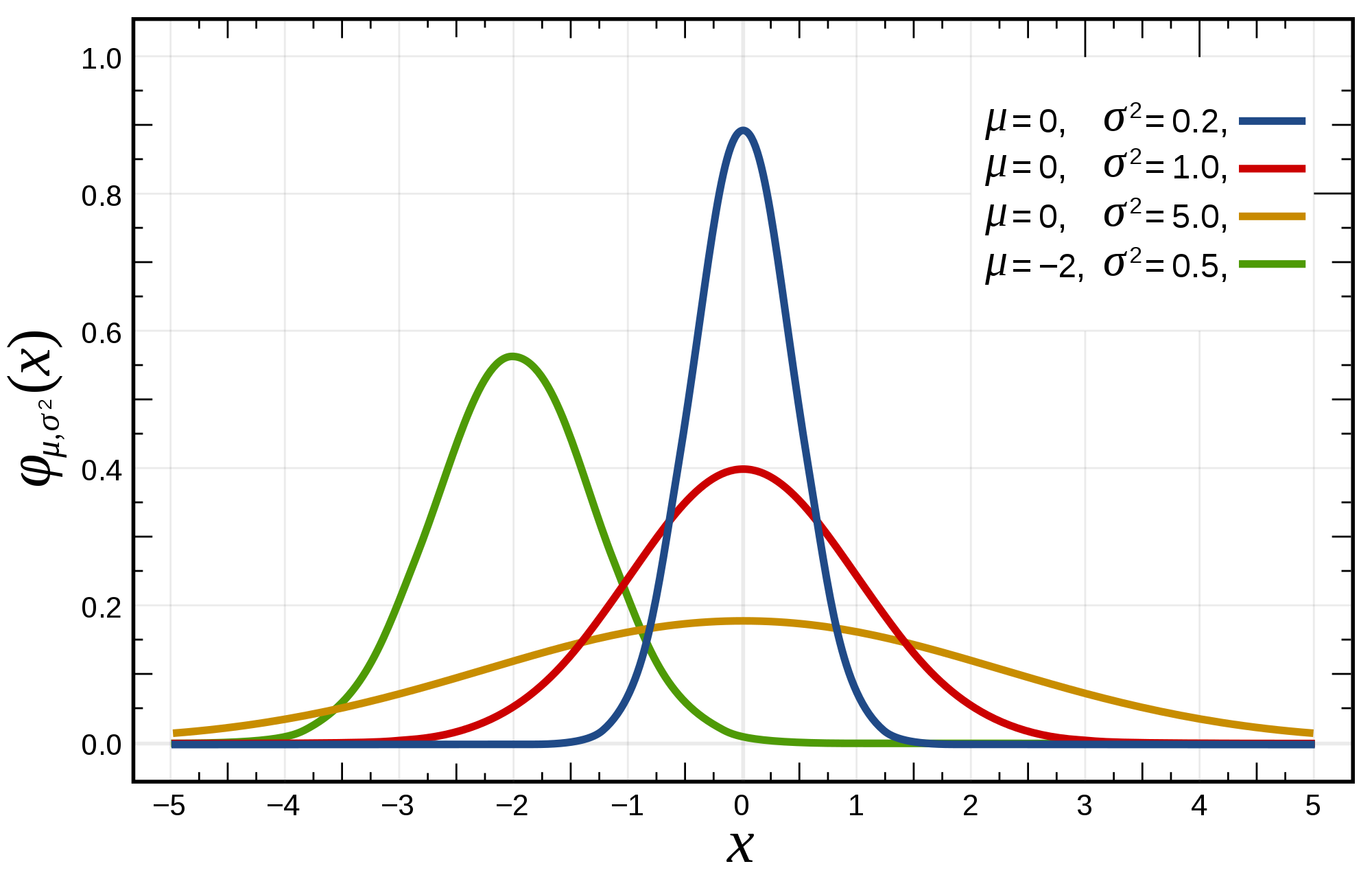## Lesson

### The big picture

Our RCT attempts to answer a research question by collecting data from a sample of a population.

Data collection consists in measuring the values of several variables for each member of the population.

First, we gain insight into the data we have collected by inspecting it, organising it, summarising it. This is descriptive statistics.

Then, we use the data to draw conclusions about the population from which the sample is collected, and to test the reliability of those conclusions. This is inferential statistics.

### Descriptive statistics

#### Variables

Variables may be continuous or discrete.

Continuous variables can be measured with arbitrary precision. Think of the age variable in our dataset: we are measuring it to the nearest year, but in theory we could measure this in seconds, nanoseconds or even more accurately.

In contrast, Discrete variables take only a fixed number of possible values. Look at the random variable in our dataset which only takes the values ‘drain’ and ‘skin’, or the satisfaction variable that takes only the values ‘Poor’, ‘Satisfactory’‘, Good’ and ‘Excellent’.

NB. The values of random don’t seem to have any particular ordering, but the values for satisfaction do: ‘Good’ is higher than ‘Satisfactory’, and so on. We therefore call this an ordinal variable. However, are we sure that the distance between ‘Poor’ and ‘Satisfactory’ is the same as that between ‘Good’ and ‘Excellent’, etc? Maybe not. We should probably conclude, then, that the satisfaction variable isn’t interval. It is worth thinking about these things because they affect the tests we can use later on.

#### Distributions

Let’s think about the age variable in our data can take, and how often it takes each one. We can visualise this with a histogram:

``````# The 'breaks=20' part dictates how many 'bins' the histogram uses
hist(RCT\$age, breaks = 20)``````

Our RCT dataset contains 64 rows of observations, but imagine if it contained 1 million rows, or even more. We would start to build up a detailed picture of how often different ages occur in our data. This would be the distribution of the age variable. Let’s look at a few of the other variables.

``````hist(RCT\$ps12)
hist(RCT\$id, breaks = 20)``````

ps12 could be described as right-tailed, or right-skewed. It might seem a bit irrelevant to plot a histogram for the id variable, but this is a great example of the uniform distribution (where all states of the variable are equally likely). There is another, very important distribution you will also have heard of:Several normal distributions

We can see how the centre-point of the bell curve shifts right and left as the mean of the distribution changes, and how the thin-ness or fatness of the distribution alters as we change the standard deviation.

A key question to ask yourself as you inspect the histograms for your data is, does this variable approximate the normal distribution? That is, if we kept taking more-and-more observations in our experiment, would the histogram we obtain look more-and-more like a bell-curve? Again, this is important to think about because if affects the tests we can use later.

It actually turns out that none of the variables in our RCT are normally distributed (age comes the closest, but it is too left-skewed). Let’s pretend for a second that the age data were normal:

``````RCT\$fake_age <- rnorm(nrow(data), mean = 50, sd = 2))
hist(RCT\$fake_age)``````

#### Means, medians and modes

Recall:

• Mean = sum of all datapoints/number of datapoints
• Median = middle datapoint, if all data were arranged in order on a line
• Mode = most common datapoint